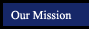Mental Math - Multiplication and Division of Whole Numbers
 Back to basic concepts What is multiplication , anyway? Factoring Positive Whole Numbers Speed practice Speed practice - How many multiplication problems in one minute? Speed practice - How many division problems in one minute?